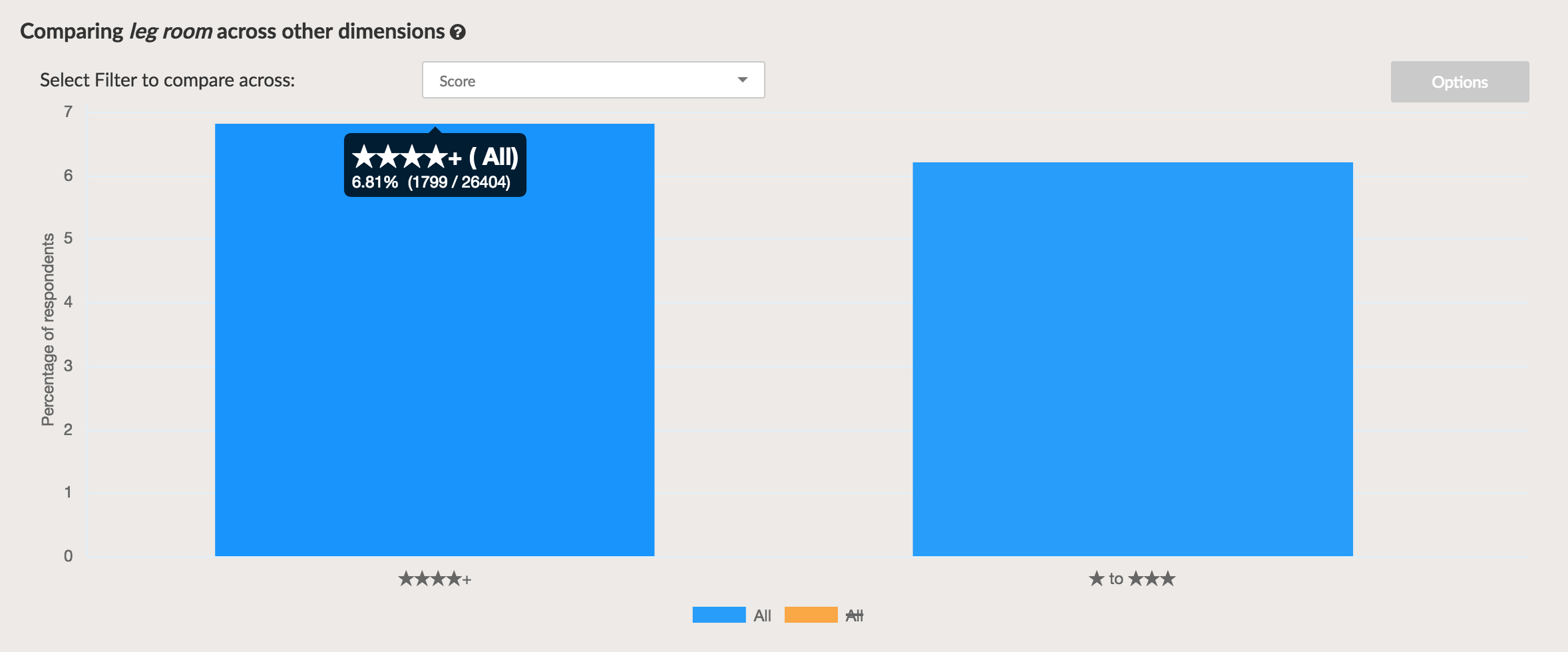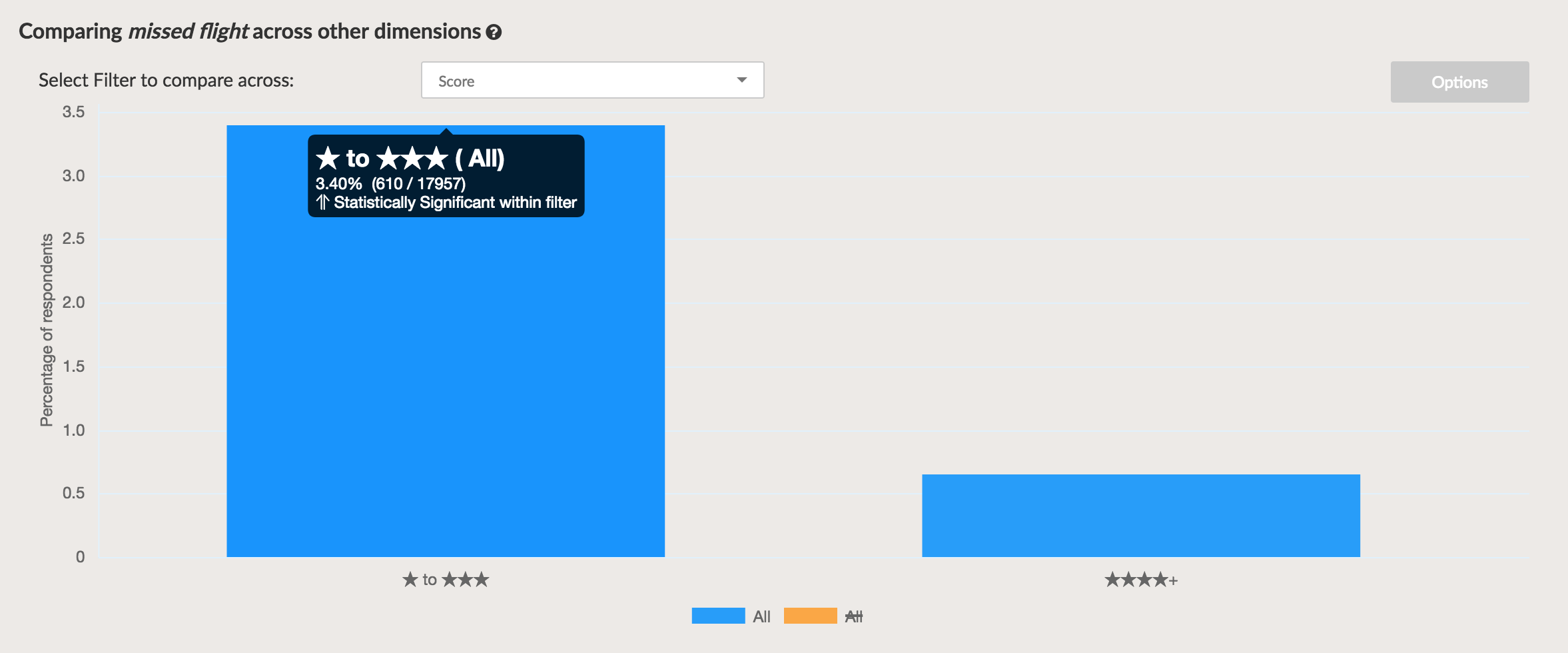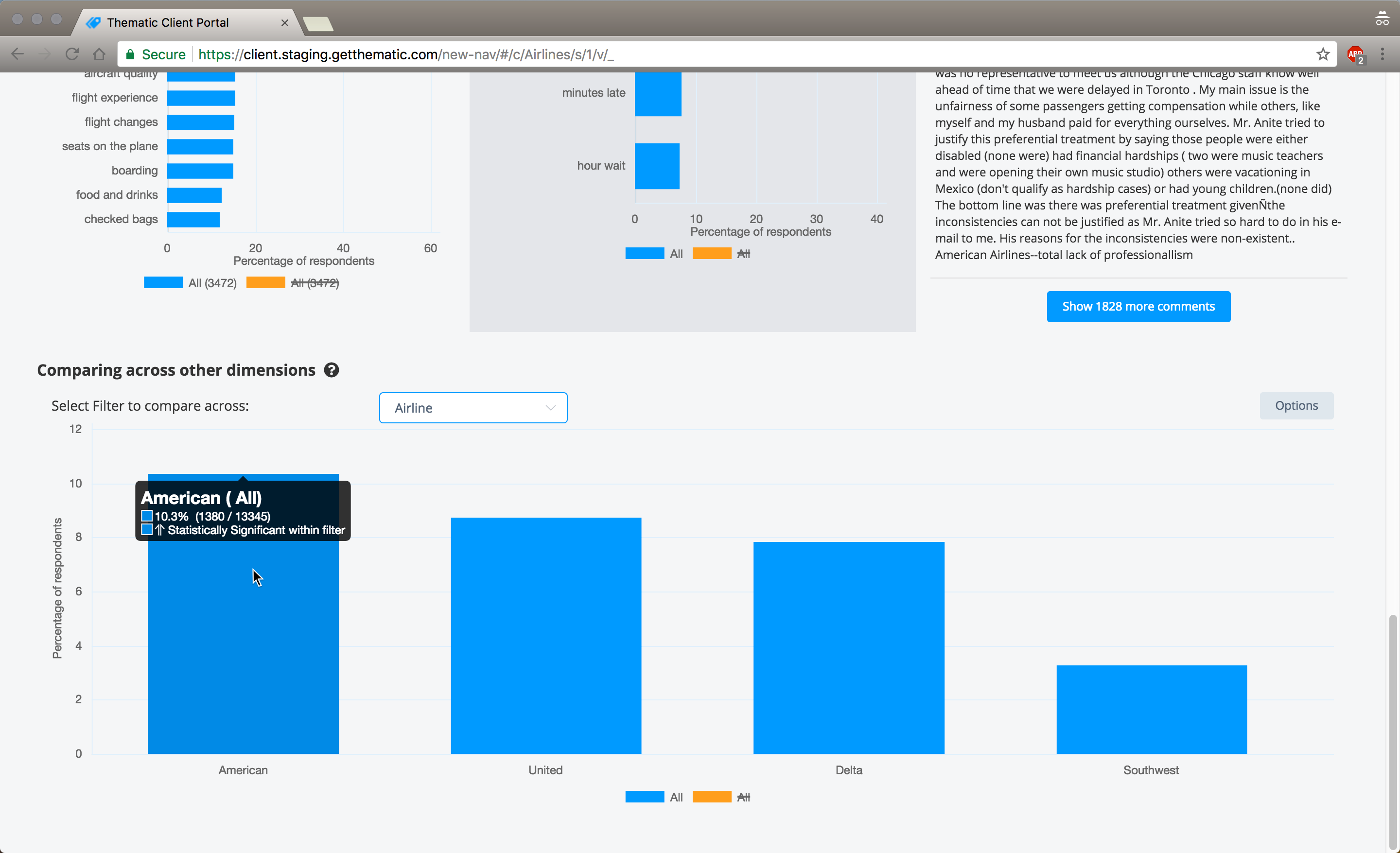# What is statistical significance?

Statistical significance is a mathematical method to determine if a result is genuine or the result of random chance.

When comparing two results using Thematic, if the difference is described as statistically significant Thematic has calculated there is a 5% or less chance that the difference is because of randomness. This means the difference can be considered genuine and not because of random chance. A statistically significant difference is more likely to create reliable insights than non-statistically significant ones.

### Examples of statistical significance

The difference in 'leg room' mentions in 4+ star reviews and 1-3 star reviews is not statistically significant. This means the difference may be caused by random chance and not genuine difference.The difference in 'missed flight' mentions between 4+ star reviews and 1-3 star reviews is statistically significant. This means the difference can be reliably assumed as genuine and not because of random chance.### When statistical significance is used in Thematic

Themes tool: A base theme in a filtered set of data with a statistically significant increase or decrease in frequency compared to the average frequency in all the data is marked with an upward or downward facing arrow.Comparison tool: If the difference in frequency of a theme in two set sets of filtered data is statistically significantly it is mentioned and marked with an upward or downward arrow.Theme analysis tool: If a theme has a statistically significant increase or decrease in frequency in a filter compared to the other filters in the filter category it is marked with an up or down arrow.